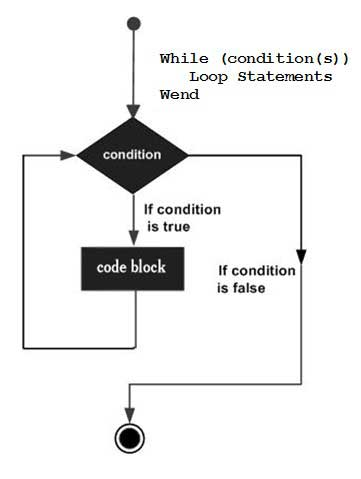# VBA - While Wend Loops

In a While…Wend loop, if the condition is True, all the statements are executed until the Wend keyword is encountered.

If the condition is false, the loop is exited and the control jumps to the very next statement after the Wend keyword.

## Syntax

Following is the syntax of a While..Wend loop in VBA.

```While condition(s)
[statements 1]
[statements 2]
...
[statements n]
Wend
```

## Flow Diagram### Example

```Private Sub Constant_demo_Click()
Dim Counter :  Counter = 10

While Counter < 15     ' Test value of Counter.
Counter = Counter + 1   ' Increment Counter.
msgbox "The Current Value of the Counter is : " & Counter
Wend   ' While loop exits if Counter Value becomes 15.
End Sub
```

When the above code is executed, it prints the following in a message box.

```The Current Value of the Counter is : 11

The Current Value of the Counter is : 12

The Current Value of the Counter is : 13

The Current Value of the Counter is : 14

The Current Value of the Counter is : 15
```
vba_loops.htm